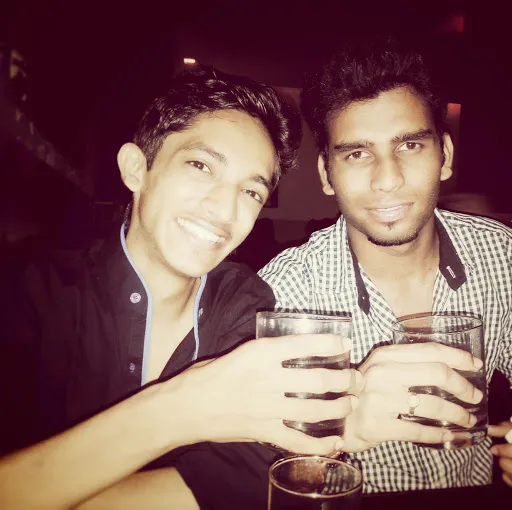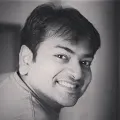# Exploring Morse Code with Idiotware shield

In this project, I am going to demonstrate how you can learn to send and receive morse code with the help of the idIoTware shield.

BeginnerFull instructions provided1 hour1,382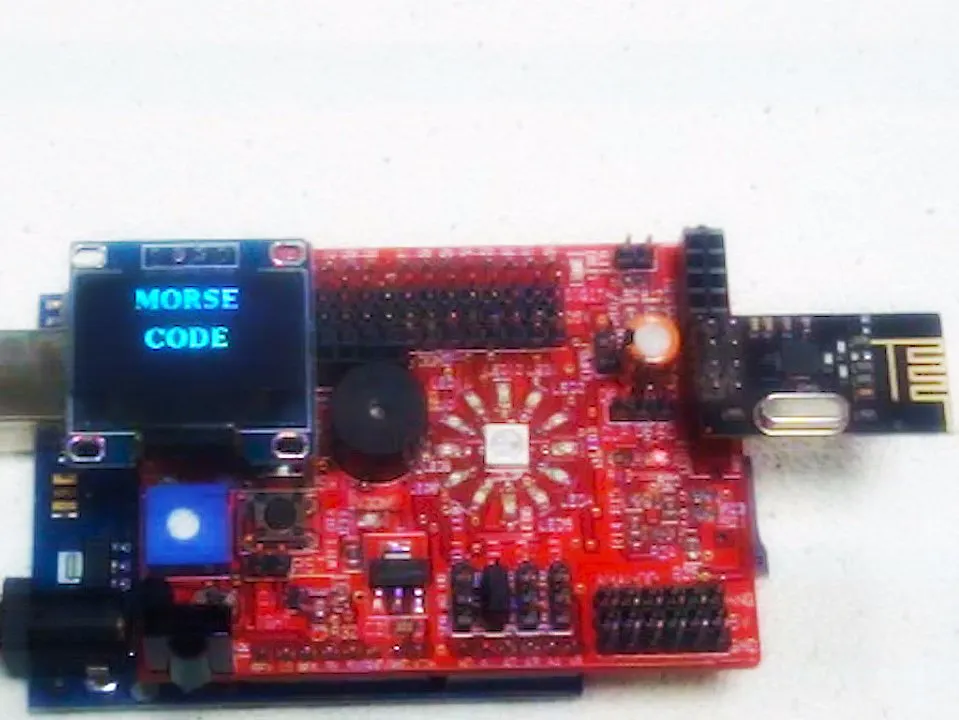## Things used in this project

### Hardware componentsArduino UNO
×2
 NRF24L01+ Wireless Module 2.4G
×2
 0.96" I2C oled display
×1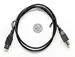USB-A to B Cable
×2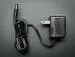9V 1A Switching Wall Power Supply
×2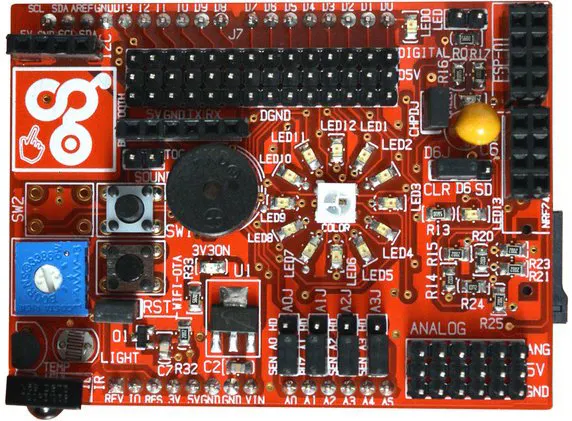idIoTware Shield
×2

### Software apps and online servicesArduino IDE

## Code

### Morse Transmitter side

Arduino
```/*
MORSE CODE

Morse code is a method of sending text messages by keying in a series of electronic pulses,
usually represented as a short pulse (called a "dot") and a long pulse (a "dash"). The code
was devised by Samuel F. B. Morse in the 1840s

In this example, we are using two NRF24L01 radio modules to transmit and receive Morse Code.
On Transmitter side, Interpret presses on button (connected to digital pin 7) as Morse Code
pattern consist of DOTs and DASHes to that particular letter and also print that letter to
OLED with its morse code.

For Example, to send letter A (DOT,DASH),
press pushbutton two times, for DOT press pushbutton for 200 milliseconds,
for DASH press pushbutton for 600 milliseconds,
time gap between DOT and DASH should be less than 200 milliseconds.
To send HELLO word

H --> (DOT,DOT,DOT,DOT)      // press button for 200 millisconds four times then wait for 600 milliseconds which
// is gap between two letters (in milliseconds), then Arduino will recognize it as letter H.
E --> (DOT)                  // then again press button for 200 milliseconds and wiat for 600 milliseconds ,then Arduino will recognize it as letter E
L --> (DOT,DASH,DOT,DOT)     // press button for 200,600,200,200 milliseconds four times respectively and wiat for 600 milliseconds,
//then Arduino will recognize it as letter L
L --> (DOT,DASH,DOT,DOT)     // press button for 200,600,200,200 milliseconds four times respectively and wiat for 600 milliseconds,
//then Arduino will recognize it as letter L
O --> (DASH,DASH,DASH)       //press button for 600,600,600 milliseconds three times and wiat for 600 milliseconds ,then Arduino will recognize it as letter 0

now to send the new word wait for 1400 milliseconds (1.4 seconds) and send particular signlas for that word

*/

#include <RF24Network.h>
#include <RF24.h>
#include <SPI.h>
#define DOT_DURATION   200               // Duration of a Morse Code "dot" (in milliseconds)
#define DASH_DURATION  DOT_DURATION * 3  // Duration of a Morse Code "dash" (in milliseconds)
#define SIGNAL_GAP     DOT_DURATION      // Gap between dots/dashes of a single letter (in ms)
#define LETTER_GAP     DOT_DURATION * 3  // Gap between two letters (in milliseconds)
#define WORD_GAP       DOT_DURATION * 7  // Gap between two words (in milliseconds)

#define BUTTON          5                // The diginal connector port to the button.

#define DOT             1                // DOT identifier
#define DASH            2                // DASH identifier
#define NONE            0                // Neither DOT nor DASH

boolean buttonWasPressed = false;        // Indicator of whether button was pressed in the last cycle
long lastTimestamp = 0;                  // Last recorded timestamp  (used for mesuring duration)
char inputSignal="";                     // Input signal buffer
int inputSignalIndex = 0;                // Index into the input signal buffer
String code = "";

const uint16_t this_node = 1;

// Address of the other node
const uint16_t other_node = 0;

boolean send_message = false;
char messageToSend = "";

void resetInputSignal()         // Reset the input signal buffer and index
{
inputSignal = NONE;
inputSignal = NONE;
inputSignal = NONE;
inputSignal = NONE;
inputSignal = NONE;
inputSignalIndex = 0;
}

void setup()
{
SPI.begin();
pinMode(BUTTON, INPUT);                // Set the button input
resetInputSignal();                    // Reset input signal buffer
Serial.begin(57600);
Serial.println("NRF24L01 MESSENGER");
}

void loop()
{ // loop forever
network.update();
if (send_message)   // if there is typed message on serial monitor ready to send
{
boolean message = network.write(header1, messageToSend, sizeof(messageToSend));   // send message to other user
if (message)
{
Serial.println("sending msg....");
Serial.print("Sender: ");   // print message on serial monitor
Serial.println(messageToSend);
send_message = false;
resetInputSignal(); // reset the input signal buffer
}

else

Serial.println("could not write....\n"); // if it is failed to send message prompt error
}
long currentTimestamp  = millis(); // get the current timestamp
long duration = currentTimestamp - lastTimestamp; // get elapsed time
{
// if the button is pressed
if (!buttonWasPressed)
{
//  if the button was previously not pressed
buttonWasPressed = true; // remember the button press
lastTimestamp = currentTimestamp; // record the time of the button press
if (duration > LETTER_GAP)
{
Serial.print(' ');
}
} // end of if (button was not pressed)
}
else
{
// the button is not pressed
if (buttonWasPressed)
{
// the button was just released
if (duration < DOT_DURATION)
{
// if the button was pressed for up to DOT cutoff
inputSignal[inputSignalIndex] = DOT; // remember the current signal as a DOT
}
else
{
// if the button was pressed for longer than DOT cutoff
inputSignal[inputSignalIndex] = DASH; // remember the current signal as a DASH
}
inputSignalIndex++; // advance the index to the input signal buffer
buttonWasPressed = false; // consume previous button press
lastTimestamp = currentTimestamp; // record the time the button was released
}
else
{
// the button was not just released
if (inputSignalIndex > 0)
{
// if there is data in the input signal buffer
if (duration > SIGNAL_GAP || inputSignalIndex >= 4)
{
// if we have a complete letter
currentInputSignalToLetter();
send_message = true;
Serial.print(messageToSend); // parse the letter and send it via serial
Serial.println(currentInputSignalToLetter());
send_message = true;
}
}
} // end of else (button was not previously pressed)
} // end of else (button is not pressed)
} // end of loop

// return true if s0-s4 match input signal
boolean matchInputSignal(byte s0, byte s1, byte s2, byte s3, byte s4)
{
return ((inputSignal == s0) &&
(inputSignal == s1) &&
(inputSignal == s2) &&
(inputSignal == s3) &&
(inputSignal == s4));
}

char currentInputSignalToLetter()
{
if (matchInputSignal(DOT, DASH, NONE, NONE, NONE))  { code ="12000"; strcpy(messageToSend, code.c_str()); return 'A'; }//A
if (matchInputSignal(DASH, DOT, DOT, DOT, NONE))    { code ="21110"; strcpy(messageToSend, code.c_str()); return 'B'; }//B
if (matchInputSignal(DASH, DOT, DASH, DOT, NONE))   { code ="21210"; strcpy(messageToSend, code.c_str()); return 'C'; }//C
if (matchInputSignal(DASH, DOT, DOT, NONE, NONE))   { code ="21100"; strcpy(messageToSend, code.c_str()); return 'D'; }//D
if (matchInputSignal(DOT, NONE, NONE, NONE, NONE))  { code ="10000"; strcpy(messageToSend, code.c_str()); return 'E'; }//E
if (matchInputSignal(DOT, DOT, DASH, DOT, NONE))    { code ="11210"; strcpy(messageToSend, code.c_str()); return 'F'; }//F
if (matchInputSignal(DASH, DASH, DOT, NONE, NONE))  { code ="22100"; strcpy(messageToSend, code.c_str()); return 'G'; }//G
if (matchInputSignal(DOT, DOT, DOT, DOT, NONE))     { code ="11110"; strcpy(messageToSend, code.c_str()); return 'H'; }//H
if (matchInputSignal(DOT, DOT, NONE, NONE, NONE))   { code ="11000"; strcpy(messageToSend, code.c_str()); return 'I'; }//I
if (matchInputSignal(DOT, DASH, DASH, DASH, NONE))  { code ="12220"; strcpy(messageToSend, code.c_str()); return 'J'; }//J
if (matchInputSignal(DASH, DOT, DASH, NONE, NONE))  { code ="21200"; strcpy(messageToSend, code.c_str()); return 'K'; }//K
if (matchInputSignal(DOT, DASH, DOT, DOT, NONE))    { code ="12110"; strcpy(messageToSend, code.c_str()); return 'L'; }//L
if (matchInputSignal(DASH, DASH, NONE, NONE, NONE)) { code ="22000"; strcpy(messageToSend, code.c_str()); return 'M'; }//M
if (matchInputSignal(DASH, DOT, NONE, NONE, NONE))  { code ="21000"; strcpy(messageToSend, code.c_str()); return 'N'; }//N
if (matchInputSignal(DASH, DASH, DASH, NONE, NONE)) { code ="22200"; strcpy(messageToSend, code.c_str()); return 'O'; }//O
if (matchInputSignal(DOT, DASH, DASH, DOT, NONE))   { code ="12210"; strcpy(messageToSend, code.c_str()); return 'P'; }//P
if (matchInputSignal(DASH, DASH, DOT, DASH, NONE))  { code ="22120"; strcpy(messageToSend, code.c_str()); return 'Q'; }//Q
if (matchInputSignal(DOT, DASH, DOT, NONE, NONE))   { code ="12100"; strcpy(messageToSend, code.c_str()); return 'R'; }//R
if (matchInputSignal(DOT, DOT, DOT, NONE, NONE))    { code ="11100"; strcpy(messageToSend, code.c_str()); return 'S'; }//S
if (matchInputSignal(DASH, NONE, NONE, NONE, NONE)) { code ="20000"; strcpy(messageToSend, code.c_str()); return 'T'; }//T
if (matchInputSignal(DOT, DOT, DASH, NONE, NONE))   { code ="11200"; strcpy(messageToSend, code.c_str()); return 'U'; }//U
if (matchInputSignal(DOT, DOT, DOT, DASH, NONE))    { code ="11120"; strcpy(messageToSend, code.c_str()); return 'V'; }//V
if (matchInputSignal(DOT, DASH, DASH, NONE, NONE))  { code ="12200"; strcpy(messageToSend, code.c_str()); return 'W'; }//W
if (matchInputSignal(DASH, DOT, DOT, DASH, NONE))   { code ="21120"; strcpy(messageToSend, code.c_str()); return 'X'; }//X
if (matchInputSignal(DASH, DOT, DASH, DASH, NONE))  { code ="21220"; strcpy(messageToSend, code.c_str()); return 'Y'; }//Y
if (matchInputSignal(DASH, DASH, DOT, DOT, NONE))   { code ="22110"; strcpy(messageToSend, code.c_str()); return 'Z'; }//Z
if (matchInputSignal(DOT, DASH, DASH, DASH, DASH))  { code ="12222"; strcpy(messageToSend, code.c_str()); return '1'; }//1
if (matchInputSignal(DOT, DOT, DASH, DASH, DASH))   { code ="11222"; strcpy(messageToSend, code.c_str()); return '2'; }//2
if (matchInputSignal(DOT, DOT, DOT, DASH, DASH))    { code ="11122"; strcpy(messageToSend, code.c_str()); return '3'; }//3
if (matchInputSignal(DOT, DOT, DOT, DOT, DASH))     { code ="11112"; strcpy(messageToSend, code.c_str()); return '4'; }//4
if (matchInputSignal(DOT, DOT, DOT, DOT, DOT))      { code ="11111"; strcpy(messageToSend, code.c_str()); return '5'; }//5
if (matchInputSignal(DASH, DOT, DOT, DOT, DOT))     { code ="21111"; strcpy(messageToSend, code.c_str()); return '6'; }//6
if (matchInputSignal(DASH, DASH, DOT, DOT, DOT))    { code ="22111"; strcpy(messageToSend, code.c_str()); return '7'; }//7
if (matchInputSignal(DASH, DASH, DASH, DOT, DOT))   { code ="22211"; strcpy(messageToSend, code.c_str()); return '8'; }//8
if (matchInputSignal(DASH, DASH, DASH, DASH, DOT))  { code ="22221"; strcpy(messageToSend, code.c_str()); return '9'; }//9
if (matchInputSignal(DASH, DASH, DASH, DASH, DASH)) { code ="22222"; strcpy(messageToSend, code.c_str()); return '0'; }//0
return '?';
}
```

Arduino
```/*
MORSE CODE

Morse code is a method of sending text messages by keying in a series of electronic pulses,
usually represented as a short pulse (called a "dot") and a long pulse (a "dash"). The code
was devised by Samuel F. B. Morse in the 1840s

In this example, we are using two NRF24L01 radio modules to transmit and receive Morse Code.
On Transmitter side, Interpret presses on button (connected to digital pin 7) as Morse Code
pattern consist of DOTs and DASHes to that particular letter and also print that letter to
OLED with its morse code.

For Example, to send letter A (DOT,DASH),
press pushbutton two times, for DOT press pushbutton for 200 milliseconds,
for DASH press pushbutton for 600 milliseconds,
time gap between DOT and DASH should be less than 200 milliseconds.
To send HELLO word

H --> (DOT,DOT,DOT,DOT)      // press button for 200 millisconds four times then wait for 600 milliseconds which
// is gap between two letters (in milliseconds), then Arduino will recognize it as letter H.
E --> (DOT)                  // then again press button for 200 milliseconds and wiat for 600 milliseconds ,then Arduino will recognize it as letter E
L --> (DOT,DASH,DOT,DOT)     // press button for 200,600,200,200 milliseconds four times respectively and wiat for 600 milliseconds,
//then Arduino will recognize it as letter L
L --> (DOT,DASH,DOT,DOT)     // press button for 200,600,200,200 milliseconds four times respectively and wiat for 600 milliseconds,
//then Arduino will recognize it as letter L
O --> (DASH,DASH,DASH)       //press button for 600,600,600 milliseconds three times and wiat for 600 milliseconds ,then Arduino will recognize it as letter 0

now to send the new word wait for 1400 milliseconds (1.4 seconds) and send particular signlas for that word

*/

#include "U8glib.h"
#include <RF24Network.h>
#include <RF24.h>
#include <SPI.h>
#define WordGap 1400
// Hardware configuration
// Set up nRF24L01 radio on SPI bus plus pins 9 & 10
U8GLIB_SSD1306_128X64 u8g(U8G_I2C_OPT_NONE|U8G_I2C_OPT_DEV_0);	// I2C / TWI

const uint16_t node_1 = 0;

// Address of the master node
const uint16_t master_node = 1;

boolean send_message = false;
char messageToSend = "";
unsigned long lastTimestamp =0;
void setup()
{
Serial.begin(57600);

// u8g.setFont(u8g_font_unifont);
u8g.setColorIndex(1); // Indataucts the display to draw with a pixel on.
Serial.println("MORSE CODE");
showMessageOnLcd(27,17,"MORSE",35,45,"CODE");
delay(1000);
SPI.begin();
}

//function to print received data on OLED
void showMessageOnLcd(int x,int y, String message1,int a,int b, String message2)
{
u8g.firstPage();
do { u8g.setFont(u8g_font_timB14);
u8g.drawStr( x, y, message1.c_str());
u8g.drawStr( a, b, message2.c_str());
u8g.setFont(u8g_font_timB12);
} while( u8g.nextPage() );
}

void loop()
{
unsigned long currentTimestamp  = millis(); // get the current timestamp
unsigned long duration = currentTimestamp - lastTimestamp; // get elapsed time
// Pump the network regularly
network.update();
if (send_message)   // if there is typed message on serial monitor ready to send
{
boolean message = network.write(header1, messageToSend, 32);   // send message to other user
if (message)
{
//Serial.print("Sender: ");   // print message on serial monitor
//Serial.println(messageToSend);
send_message = false;
}

else

Serial.println("could not write \n"); // if it is failed to send message prompt error
showMessageOnLcd(1,15,"could not write",5,45,"");
}

// Is there anything ready for us?
while (network.available() )
{ // If so, grab it and print it out
char messageToRecieve = "";
boolean recieve = false;
while (!recieve)
{
Serial.print("Reciver: ");   // print recived data on serial monitor
Serial.println(messageToRecieve);
lastTimestamp = currentTimestamp;
}
String MorseCode(messageToRecieve);    // convert char array of received message to string
Serial.println(showLetter(MorseCode)); //and print letter on serial monitor
showLetter(MorseCode);       // show letter on OLED
if (duration > WordGap)      //if delay between received messages is greater than WordGap(1400)
{                         // add space between words
}
if (receivedWord.length()>=13)  // if stringlenth is greater than 13, empty the string
{
}
}

}

// function to decode the received morse code to respective letter and print that letter on OLED
char showLetter(String data)
{
if (data == "12000") { showMessageOnLcd(5,13,"A",25,15,"._");       return 'A';}
if (data == "21110") { showMessageOnLcd(5,13,"B",25,15,"_...");     return 'B';}
if (data == "21210") { showMessageOnLcd(5,13,"C",25,15,"_._.");     return 'C';}
if (data == "21100") { showMessageOnLcd(5,13,"D",25,15,"_..");      return 'D';}
if (data == "10000") { showMessageOnLcd(5,13,"E",25,15,".");        return 'E';}
if (data == "11210") { showMessageOnLcd(5,13,"F",25,15,".._.");     return 'F';}
if (data == "22100") { showMessageOnLcd(5,13,"G",25,15,"_ _.");     return 'G';}
if (data == "11110") { showMessageOnLcd(5,13,"H",25,15,"....");     return 'H';}
if (data == "11000") { showMessageOnLcd(5,13,"I",25,15,"..");       return 'I';}
if (data == "12220") { showMessageOnLcd(5,13,"J",25,15,"._ _ _");   return 'I';}
if (data == "21200") { showMessageOnLcd(5,13,"K",25,15,"_._");      return 'K';}
if (data == "12110") { showMessageOnLcd(5,13,"L",25,15,"._..");     return 'L';}
if (data == "22000") { showMessageOnLcd(5,13,"M",25,15,"_ _");      return 'M';}
if (data == "21000") { showMessageOnLcd(5,13,"N",25,15,"_.");       return 'N';}
if (data == "22200") { showMessageOnLcd(5,13,"O",25,15,"_ _ _");    return 'O';}
if (data == "12210") { showMessageOnLcd(5,13,"P",25,15,"._ _.");    return 'P';}
if (data == "22120") { showMessageOnLcd(5,13,"Q",25,15,"_ _._");    return 'Q';}
if (data == "12100") { showMessageOnLcd(5,13,"R",25,15,"._.");      return 'R';}
if (data == "11100") { showMessageOnLcd(5,13,"S",25,15,"...");      return 'S';}
if (data == "20000") { showMessageOnLcd(5,13,"T",25,15,"_");        return 'T';}
if (data == "11200") { showMessageOnLcd(5,13,"U",25,15,".._");      return 'U';}
if (data == "11120") { showMessageOnLcd(5,13,"V",25,15,"..._");     return 'V';}
if (data == "12200") { showMessageOnLcd(5,13,"W",25,15,"._ _");     return 'W';}
if (data == "21120") { showMessageOnLcd(5,13,"X",25,15,"_.._");     return 'X';}
if (data == "21220") { showMessageOnLcd(5,13,"Y",25,15,"_._ _");    return 'Y';}
if (data == "22110") { showMessageOnLcd(5,13,"Z",25,15,"_ _..");    return 'Z';}
if (data == "12222") { showMessageOnLcd(5,13,"1",25,15,"._ _ _ _"); return '1';}
if (data == "11222") { showMessageOnLcd(5,13,"2",25,15,".._ _ _ "); return '2';}
if (data == "11122") { showMessageOnLcd(5,13,"3",25,15,"..._ _");   return '3';}
if (data == "11112") { showMessageOnLcd(5,13,"4",25,15,"...._");    return '4';}
if (data == "11111") { showMessageOnLcd(5,13,"5",25,15,".....");    return '5';}
if (data == "21111") { showMessageOnLcd(5,13,"6",25,15,"_....");    return '6';}
if (data == "22111") { showMessageOnLcd(5,13,"7",25,15,"_ _...");   return '7';}
if (data == "22211") { showMessageOnLcd(5,13,"8",25,15,"_ _ _..");  return '8';}
if (data == "22221") { showMessageOnLcd(5,13,"9",25,15,"_ _ _ _."); return '9';}
if (data == "22222") { showMessageOnLcd(5,13,"0",25,15,"_ _ _ _ _");return '0';}
}
```

## Credits

### Ravi Parmar

13 projects • 40 followers
I am an Electronics and telecom graduate engineer with passion in embedded technology and making new stuff .

### Rupin Chheda

35 projects • 80 followers
Resident Maker at CuriosityGym! Electronics Engineer, CAD Modeller, Educator